×

### Html & Html5

Introduction to Html

Html5 Grid

Html5 Graphics

Html5 Media

### Loops in Python

Loops are the repetition of any statement or block of statement . Here also loops are used in Python
And Python has two types of loop commands :-

• while loops
• for loops

Let’s understand these in a detailed manner :-

### Python while loops

while loop repeatedly executes a target statement as long as a given condition is true .

``` Syntax :     while expression
statement(s)```

Statement is a single block statement .The condition may be any expression and true is any non-zero value.The loop intrates while the condition is true.

Flow diagram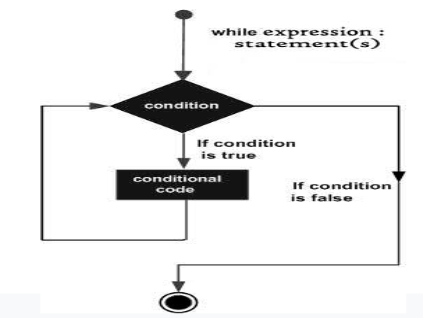### Program while loop

Let’s understand this by the following example :-

a = 1

while a<5 :

print(a)

a += 1

### Output of this ProgramSo here we just get the numbers from 1 to 4 by using the while loop.

the break Statement

With break statement we can stop the loop even if while loop is true.
Example:

i = 1

while i < 5:

print(i)

if (i == 3):

break

i += 1

### Output of this Program

Here in the above program firstly we gave command to our Python interpreter that gives us the continuous number from 1 to 5 , than through break statement we just make him stop as soon as it reach upto number 3 .
Let’s see what’s happens :-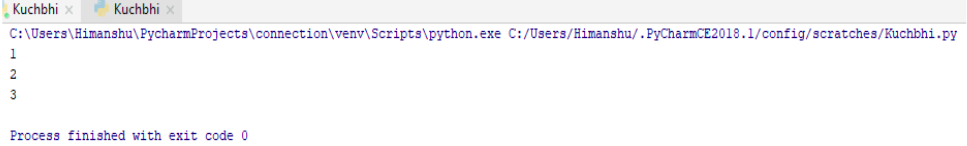So it just gave us the desired output.

the continue Statement

With the continue statement we can stop the current iteration and continue the next .

Example:

i = 0

while i < 5:

i += 1

if (i == 3):

continue

print(i)

### Output of this Program

Here in the above program firstly we gave command to our Python interpreter that gives us the continuous number from 1 to 5 , than through continue statement we just skip the loop number 3 .
Let’s see what’s happens :-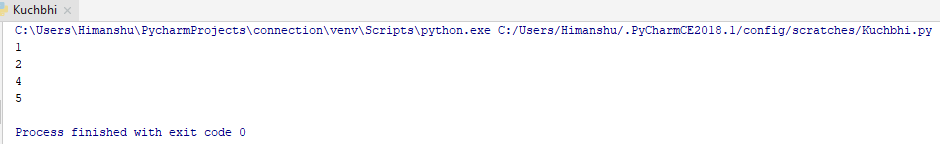So here you can see we get all except 3 because we just skip it .

### Python for loops

For loop we have also observed in our collection data type . It is basically used for the iteration over our collection data type .

We can use ‘for’ loop in our list data type , string data type , set data type , dictionary data type and for loops with break or continue statement.
Ok so let’s understand these by the example :-

for loops in List

Looping in list through for keyword.

Example:

thislist = ['Fruits', 'Vegetables', 'Sports']

for x in thislist :

print(x)

### Output of this ProgramHere all list items we get one by one.

for loops in String

As we know String contains the characters . So if we want to get any sequence of characters present in string, we can also perform it here.

Example:

for x in "Manish" :

print(x)

### Output of this Program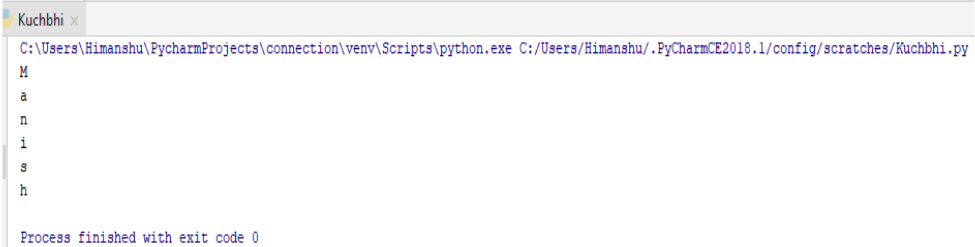We just get our characters in a sequence .

break statement in for loop

Here also in for loop we can exit or come out of our loop just by the break keyword.

Example:

color= ['Red', 'Blue' ,'White']

for x in color :

print(x)

if x=='Blue':

break

### Output of this Program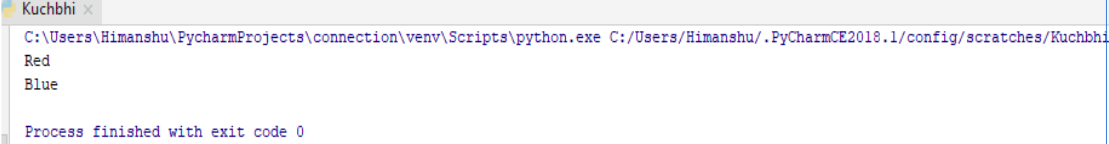So break statement breaks the loop after ‘Blue’.

continue statement in for loop

Here also in any loop if we can stop the current iteration but follows next one by the continue keyword.

Example:

color= ['Red', 'Blue' ,'White']

for x in color :

if x=='Blue':

continue

print(x)

### Output of this Program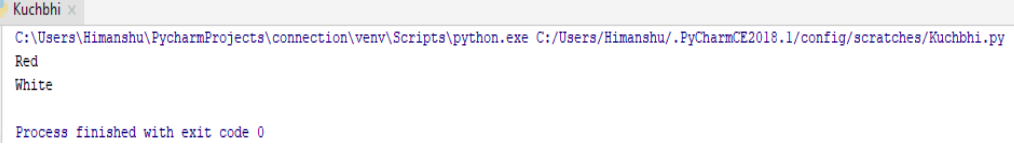It just didn’t print ‘Blue’ because we just skip that with our continue keyword.

range () function

This function represents a sequence of numbers starting from 0 (by default) to any specified number

(a) range(8) - it means the sequence is from 0 to 7 not 0 to 8

Example:

for x in range(5) :

print(x)

### Output of this Program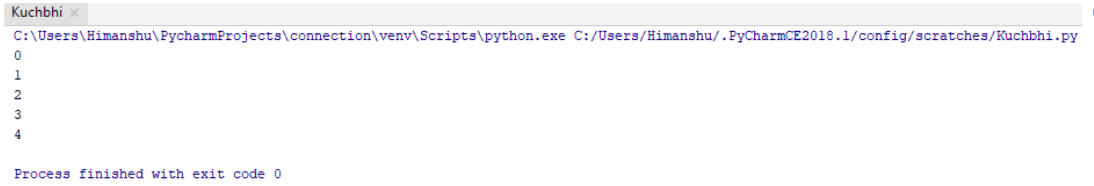Just by the range function we have get the numbers from 0 to 7 .

(b) By default the range starts from ‘0’ but if we want to specify or want to change the initial point than we can also do that .
Let’s understand this by an example :-

for x in range(3,8):

print(x)

### Output of this Program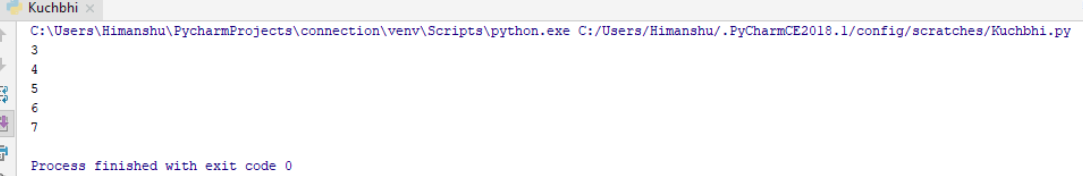So here also we get our desired value

(c) By default in range function it increments by one but if you want to change the increment value , yes it is possible. We have to add third parameter to do so .
Let’s understand this by an example :-

for x in range(3,8,2):

print(x)

### Output of this Program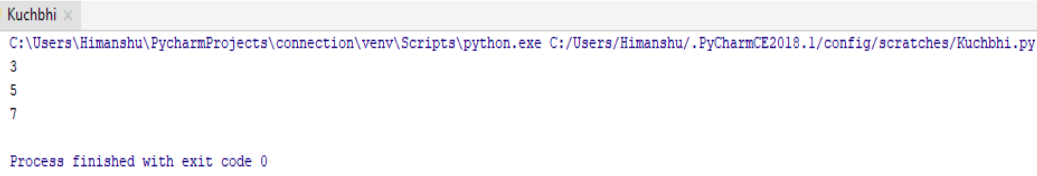So it just gave us the desired output. By default it increments 1 .

### Nested loops

When we use loop inside loops . Than also we can take help of ‘for’ keyword.
Let’s take an example :-

fruits=['Apple', 'Litchi', 'Mango']

color=['Red','Yellow', 'Orange']

for x in fruits:

for y in color:

print(x,y)

### Output of this Program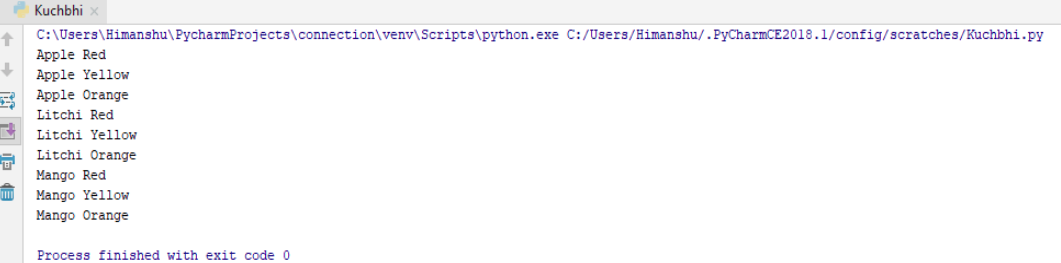So here we get our both loops in the iteration

python training insitute| Best IT Training classes in Gurgaon | python training